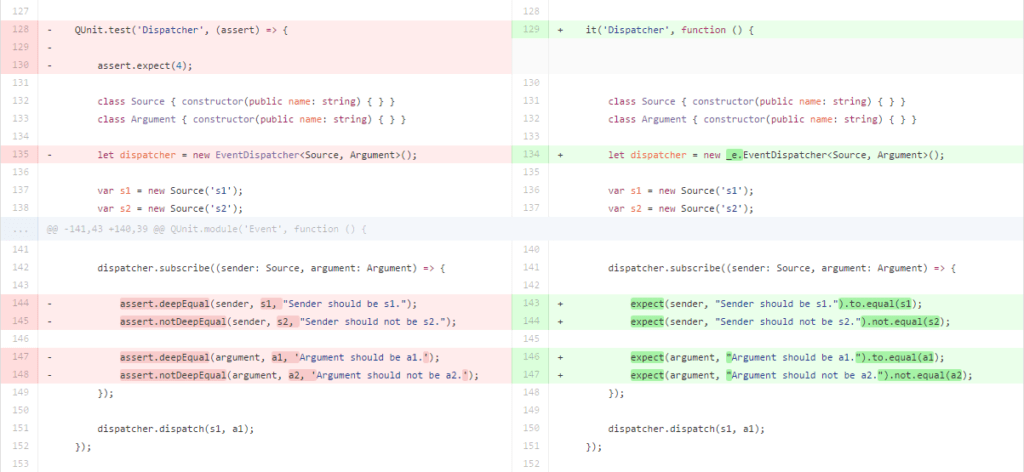Recently I’ve been playing around with NPM. I switched my unit tests from QUnit to Mocha. This was not as straight forward as one would hope. In this blog I’ll show some example code. At the end I’ll link to a side by side comparison of the entire test project. Hopefully it helps you to convert your code. I’ll be using the Chai BDD assertion engine.

## QUnit example

Let’s take a look at a QUnit test. It has a module to split your tests up into functional parts. The test method is used to bundle a test. One test can contain one or more asserts to do the actual testing.

```QUnit.module('Event', function () {

QUnit.test("Event list", (assert) => {

assert.expect(4);

var events = new EventList<any, string>();
var result: string;

events.get('Test1').subscribe((sender: any, args: string) => result = args);
events.get('Test1').dispatch(this, 'Testing 123');

assert.equal(result, 'Testing 123', 'The result should be "Testing 123".');

events.get('Test2').dispatch(this, 'Testing 456');
assert.equal(result, 'Testing 123', 'The result should still be "Testing 123".');

events.get('Test2').subscribe((sender: any, args: string) => result = args);
events.get('Test2').dispatch(this, 'Testing 789');
assert.equal(result, 'Testing 789', 'The result should be "Testing 789".');

events.get('Test3').asEvent().subscribe((sender: any, args: string) => result = args);
events.get('Test3').dispatch(this, 'Testing 42');
assert.equal(result, 'Testing 42', 'The result should be "Testing 42".');
});
});```

## Mocha me!

Let’s convert the example into Mocha / Chai. The module can be translated into a describe. The it specifies the test. Note that the execution of the it’s are not synchronous! Each test can be rewritten using expect and to.equal or not.equal.

```describe('Event', function () {

it("Event list", function () {

var events = new EventList<any, string>();
var result: string;

events.get('Test1').subscribe((sender: any, args: string) => result = args);
events.get('Test1').dispatch(this, 'Testing 123');
expect(result, 'The result should be "Testing 123".').to.equal('Testing 123');

events.get('Test2').dispatch(this, 'Testing 456');
expect(result, 'The result should still be "Testing 123".').to.equal('Testing 123');

events.get('Test2').subscribe((sender: any, args: string) => result = args);
events.get('Test2').dispatch(this, 'Testing 789');
expect(result, 'The result should be "Testing 789".').to.equal('Testing 789');

events.get('Test3').asEvent().subscribe((sender: any, args: string) => result = args);
events.get('Test3').dispatch(this, 'Testing 42');
expect(result, 'The result should be "Testing 42".').to.equal('Testing 42');
});
});```

Fortunately its not that hard to convert QUnit into Mocha if you use the right conversion.

## Async – QUnit

What about asynchronous tests? Observe the following test:

```QUnit.test('Async dispatch', (assert) => {

assert.expect(2);

let done = assert.async();
let dispatcher = new EventDispatcher<any, number>();

let i = 0;

dispatcher.subscribe((s, a) => {
i = a;
assert.equal(i, 1, 'i should be 1.');
done();
});

dispatcher.dispatchAsync(null, 1);
assert.equal(i, 0, 'Because of async dispatch, i should be 0.');
});```

## Async – Mocha

Now let’s rewrite it. Plugging a function(done) { } into the it method, will cause Mocha to thread the tests as asynchronous. Invoke done when the async test is ready.

```describe('Event', function () {

it('Async dispatch', function (done) {

let dispatcher = new EventDispatcher<any, number>();

let i = 0;

dispatcher.subscribe((s, a) => {
i = a;
expect(i, 'i should be 1.').to.equal(1);
done();
});

dispatcher.dispatchAsync(null, 1);
expect(i, 'Because of async dispatch, i should be 0.').to.equal(0);
});
});```

## Side by side comparison

To make things clearer I’ve put the conversion for the Strongly Typed Events project to a Github Gist comparison:## Wrap up

My biggest mistake was using it the wrong way. Just use the following table when converting your tests:[table “” not found /]